Data Science | AI | Machine Learning | Deep Learning | Programming | Bioinformatics

# Data Visualization with Pandas in Action (Part 2)

Data visualization is one of the most enjoyable stages of data analysis. You can draw graphics very easily with the recently developed open source and free libraries. The Pandas library is one of the most used Python libraries for data preprocessing and data cleaning. Libraries such as Matplotlib and Seaborn are often used to visualize data. But with Pandas, you can easily visualize Series and DataFrame data.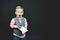Photo by Ben White on Unsplash

In my last article, I explained the bar, histogram and box graphics with Pandas.

• Area charts
• Scatter plots
• Hexagonal bin charts
• Pie…

# Data Visualization with Pandas in Action

As a data scientist, one of my favorite stages of data analysis is data visualization. When I visualize data, I feel like an artist. Here you can watch how masterpieces can be created with data visualization and machine learning.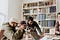Photo by Priscilla Du Preez on Unsplash

Data visualization is one of the important steps of data analysis. To visualize data, most people usually use Matplotlib and Seaborn. Pandas is one of Python’s most important libraries used for data preprocessing and data cleaning. You can also easily visualize Series and DataFrames with methods in Pandas. In my last article, I talked about scatter plots with Matplotlib in Python

# Scatter Plot with Matplotlib in Python

Data visualization is one of the first stages of data analysis. In my last post, I explained histograms with Matplotlib in Python. I will talk about the scatter plots in this post.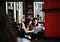Photo by Ilyuza Mingazova on Unsplash

A Scatter plot can be plotted to see the relationship between two variables. In the scatter plot, the observation values are shown with dots. If these points are spread around a line, it means that there is a relationship between the variables. Scatter plot also called a scatterplot, scatter graph, scatter chart, scattergram, or scatter diagram.

# Bar Plot with Matplotlib in Python

Suppose you have average salary data for five different jobs and you want to compare these salaries. You can use bar plots to visualize this data. Bar plots are used to compare values in different categories. Bars can be plotted horizontally or vertically. In my last article, I explained histograms with Matplotlib in Python. In this article, I will describe the bar plot.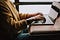Photo by Christin Hume on Unsplash

In summary, I will explain the following topics in this article.

• How to plot bar plots?
• How to plot error bars?
• How to plot bar graphs with error bars?

Before starting the topic, our Medium page includes…

# Histograms with Matplotlib in Python

You may have heard of histograms or seen in data analysis. If you are not good at statistics, you can confuse the histograms with the bar graphs. In my last post, I talked about line plots in python using matplotlib. Histograms are also similar to bar charts. But there are some differences.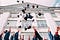Photo by Vasily Koloda on Unsplash

A histogram is used to summarize continuous or discrete data. For example, you have the notes of the introductory computer science course of the students of X universities. You want to see which grade has been taken the most, with less than average grade fields or more grade…

# Line Plots in Python using Matplotlib

Data visualization is one of the important steps of data analysis. By visualizing the data, we can explore the information in the data. One of the simplest graphs is line plots. In my last post, I explained how to plot statistical graphs with Seaborn and Pandas. In this post, I will talk about how to plot line graphs with the Matplotlib.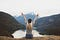y Becca Tapert on Unsplash

In summary, I will mention the following topics in this post,

• What are the line plots?
• How to plot a simple line graph?
• How to specify the color and style of the line plot?
• How to fill the area…

# Data Visualization with Pandas and Seaborn 🎓

Visualizing data is one of the important steps of data analysis. I explained the Seaborn library in the last post. In this post, I will talk about data visualization with Pandas and Seaborn. Matplotlib library and plot method in Pandas are not enough to plot advanced graphics. In order to visualize the data better and easier, Seaborn library is used, which works on the Matplotlib library and is compatible with Pandas.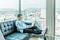Photo by Austin Distel on Unsplash

Many statistical graphs are easily plotted with Seaborn. In summary, I will mention the following topics in this post,

• Line plots
• Bar plots
• Histogram and density plots
• Scatter plots

# Seaborn Tutorial: Statistical Data Visualization

Before data analysis, the data is plotted to recognize the data. Usually, Matplotlib or Seaborn libraries are used in Python to visualize data. In my last article, I explained the Matplotlib library. In this article, I will tell about the Seaborn library.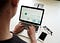Photo by Campaign Creators on Unsplash

Matplotlib is an important library for visualizing data. But we cannot plot advanced graphics using this library alone. Matplotlib library has some shortcomings. Matplotlib has low-level APIs and requires more code for advanced graphics. Another problem of Matplotlib emerged about 10 years before Pandas, so it was not designed to use DataFrame, an important data structure of Pandas.

# Matplotlib Tutorial for Beginners 📈

In the last post, I explained how to load the Matplotlib library and how to simply draw and save a graphic. In this post, I will explain how to draw graphics with Matplotlib. Before the data is analyzed, the data is visualized to recognize the data. Matplotlib is one of the most frequently used libraries in Python to visualize data.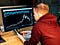Photo by Chris Liverani on Unsplash

In this post, he will briefly describe the following topics.

• How to create a graphic object?
• How to specify color, markings and line styles?
• How to adjust thickness, label and legend?
• How to add text and shape to the graph?

# Introduction to Matplotlib 📊

In my last post, I mentioned important methods for time series in Pandas library. I will talk about Matplotlib library in this post. A graphic sometimes means thousands of words. Data visualization is one of the important stages of data analysis. For example, graphs help us see outliers or build models.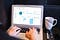Photo by Myriam Jessier on Unsplash

Matplotlib is a library used in Python to visualize data. In summary, I will explain the following topics in this lesson.

• What is Matplotlib?
• How to install Matplotlib?
• Interactive and static graphics
• Saving the graph

Before starting the topic, our Medium page includes posts on data science, artificial intelligence…